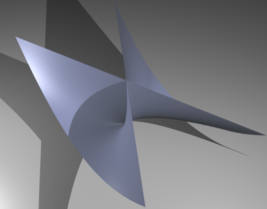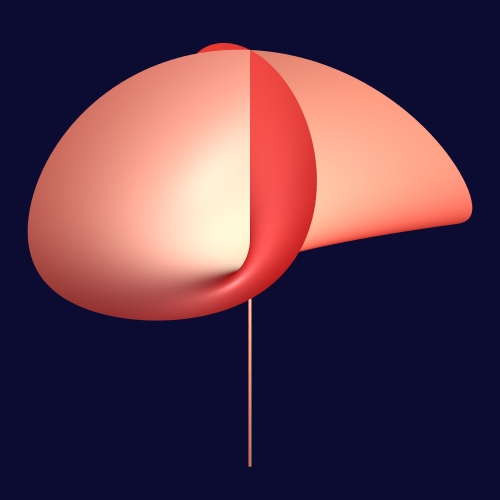## Section: Research Program

### Non-linear computational geometry

Figure 1. Two views of the Whitney umbrella (on the left, the “stick” of the umbrella, i.e., the negative $z$-axis, is missing). Right picture from [Wikipedia], left picture from [Lachaud et al.].As mentioned above, curved objects are ubiquitous in real world problems and in computer science and, despite this fact, there are very few problems on curved objects that admit robust and efficient algorithmic solutions without first discretizing the curved objects into meshes. Meshing curved objects induces a loss of accuracy which is sometimes not an issue but which can also be most problematic depending on the application. In addition, discretization induces a combinatorial explosion which could cause a loss in efficiency compared to a direct solution on the curved objects (as our work on quadrics has demonstrated with flying colors , , , , ). But it is also crucial to know that even the process of computing meshes that approximate curved objects is far from being resolved. As a matter of fact there is no algorithm capable of computing in practice meshes with certified topology of even rather simple singular 3D surfaces, due to the high constants in the theoretical complexity and the difficulty of handling degenerate cases. Even in 2D, meshing an algebraic curve with the correct topology, that is in other words producing a correct drawing of the curve (without knowing where the domain of interest is), is a very difficult problem on which we have recently made important contributions , , .

It is thus to be understood that producing practical robust and efficient algorithmic solutions to geometric problems on curved objects is a challenge on all and even the most basic problems. The basicness and fundamentality of two problems we mentioned above on the intersection of 3D quadrics and on the drawing in a topologically certified way of plane algebraic curves show rather well that the domain is still in its infancy. And it should be stressed that these two sets of results were not anecdotical but flagship results produced during the lifetime of the Vegas team.

There are many problems in this theme that are expected to have high long-term impacts. Intersecting NURBS (Non-uniform rational basis spline) in a certified way is an important problem in computer-aided design and manufacturing. As hinted above, meshing objects in a certified way is important when topology matters. The 2D case, that is essentially drawing plane curves with the correct topology, is a fundamental problem with far-reaching applications in research or R&D. Notice that on such elementary problems it is often difficult to predict the reach of the applications; as an example, we were astonished by the scope of the applications of our software on 3D quadric intersection (QI: http://vegas.loria.fr/qi/.) which was used by researchers in, for instance, photochemistry, computer vision, statistics and mathematics.